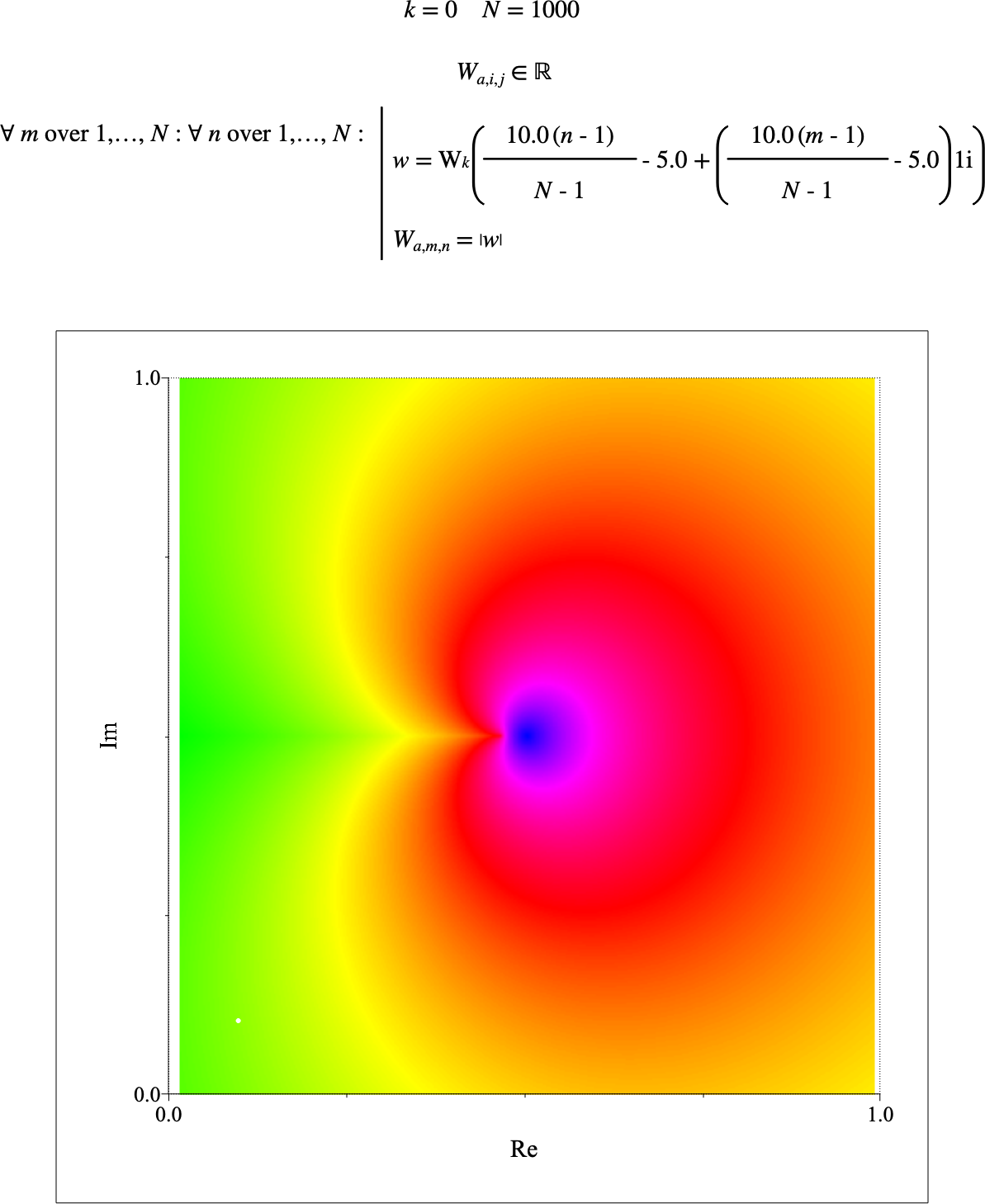# $$\text{W}$$¶

You can use the Lambert W function, $$\text{W}$$, to calculate the inverse if the function $$z = x e ^ x$$.

You can use the \lambertw backslash command to insert this function.

• $$\text{complex } \text{W} _ \text{<k>} \left ( \text{<z>} \right )$$

• $$\text{complex } \text{W} _ \text{<k>} \left ( \text{<z>}, \text{<relative error>} \right )$$

The $$k$$ parameter indicates the branch to calculate the Lambert W function over. The $$z$$ term represents the value to calculate the result over. The $$\text{<relative error>}$$ term is used to determine the allowed tolerance when Householder method is applied during the computation. The $$\text{<relative error>}$$ term can be used to speed up the calculation of the result. If not specified, a value of $$4\mathit{E}$$ will be used for the tolerance.

Aion uses either a modified version of the algorithm described by  or the faster but more limited Boost C++ library implementation which is based on the algorithm described by .

The Boost Fukushima algorithm is used when $$z > -\frac{1}{e}$$ and real, and when $$k = 0$$ or $$k = -1$$. For values where $$z$$ is close to $$-\frac{1}{e}$$, the Fukushima algorithm may not be able to resolve a value. When this occurs the Aion implementation will fall-back on the Corless algorithm.

The Corless algorithm is used when $$z$$ is complex or when the range of parameters makes the Boost Fukushima algorithm unusable.

The Aion Coreless algorithm calculates an initial guess value by one of three methods documented in .

• A series expansion about the branch point.

Figure 215 shows the basic use of the $$\text{W}$$ function.